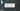# Two Sum Problem - Leet Code Solution

July 08, 2019## Problem Statement

Given an array of integers, return indices of the two numbers such that they add up to a specific target.

You may assume that each input would have exactly one solution, and you may not use the same element twice.

Example:

``````Given nums = [2, 7, 11, 15], target = 9,

Because nums + nums = 2 + 7 = 9,
return [0, 1].``````

## Solution

### Brute force solution, O(n^2)

Simply iterate over array 2 times, and look if the sum equals to target.

``````public int[] twoSum(int[] nums, int target) {
int l = nums.length;
for (int i=0; i<l; i++) {
for (int j=i+1; j<l; j++) {
if (nums[i] + nums[j] == target) {
return new int[]{i, j};
}
}
}

return null;
}``````

### Optimized approach, using HashMap/HashSet

Whenever this kind of problem comes, one thing came in mind is using HashSet. But, this problem is not asking you to return the values that sum upto a target value. It is asking you to return indexes of numbers.

So, you have to maintain a HashMap<Integer, Integer>, which Key is the number itself, and value is its index in the original array.

HashMap has the property of searching its key and value in O(1).

While iterating, we just have to check whether HashMap has the remaining difference. If its there, its a match. Else, keep a copy of this value and index in HashMap.

For this purpose, we are keeping a copy of array values and its index into a HashMap.

``````public int[] twoSum(int[] nums, int target) {
Map<Integer, Integer> hashMap = new HashMap<>();
int l = nums.length;
for (int i=0; i<l; i++) {
int pairValue = target-nums[i];
if (hashMap.containsKey(pairValue)) {
return new int[]{i, hashMap.get(pairValue)};
}

if (!hashMap.containsKey(nums[i])) {
hashMap.put(nums[i], i);
}
}

return null;
}``````